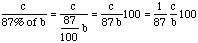Subject: Re-Percentaging Name: Doug Question: My mind has gone blank. I have a series of percentages which have been establised by the same base number. I need to remove one of the percentages (13%) leaving me with 87%. I need to arifically inflate the remaining percentages to total 100%. Any help would be appreciated. Thanks in advance, Doug Hi Doug You originally had a base number, b, of items divided into categories. If some particular category had c items in it then the percentage in that category is (c/b)x100. Now you want to remove one of the categories that has 13% of the items so the resulting new base number is 87% of b, that is (87/100)xb. The resulting fraction of the items in this category is nowThat is the new percentage in this category is the original percentage divided by 87. Cheers Penny

Go to Math Central